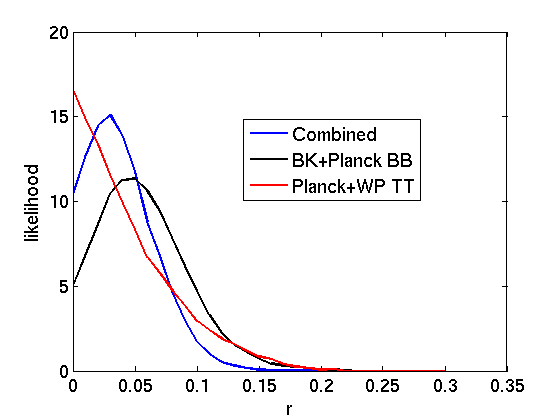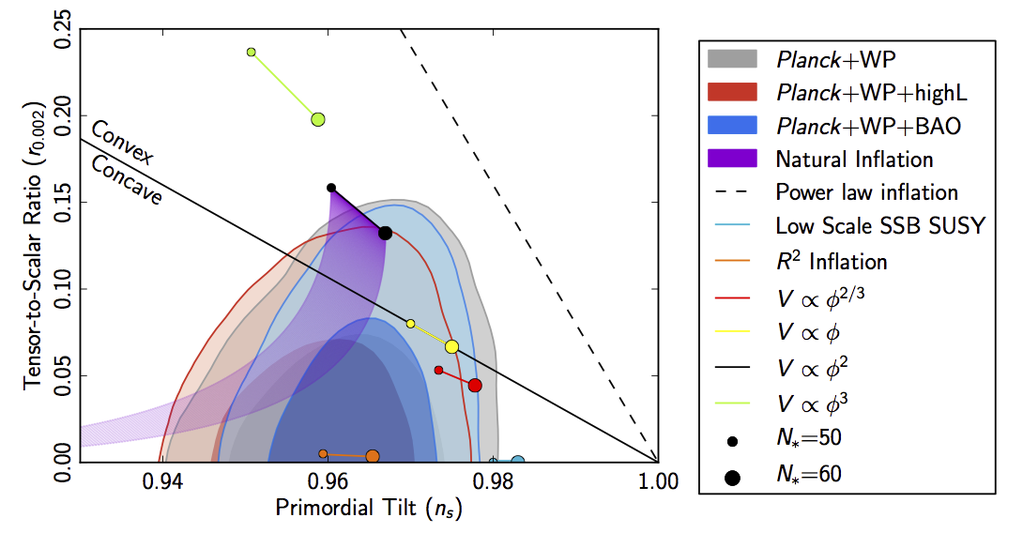## Tuesday, February 3, 2015

### Combined constraints from BICEP2, Keck, Planck and WMAP on primordial gravitational waves

This week, the joint analysis of BICEP2 (+ BICEP2's successor Keck) and Planck has finally arrived. The result is more or less what was expected, which is that what BICEP2 saw last year in the B-mode polarisation signal of the CMB was not actually primordial gravitational waves (as had originally been hoped and claimed), but was unfortunately actually due to dust in the Milky Way. Such is life. Though we did of course have the best part of a year to come to grips with this reality.Combined constraint on $$r$$ from polarisation and temperature measurements (in blue). Freshly digitised in the spirit of modern cosmology. Gives $$r\lesssim 0.09$$ at $$95\%$$ confidence.

As a result of subtracting the dust component in BICEP2/Keck's signal (obtained by comparing the measurements from BICEP2/Keck and Planck), the final constraint on the "tensor to scalar ratio" (or $$r$$) from the BICEP2/Keck measurement is that $$r<0.12$$ at $$95\%$$ confidence. This $$r$$ parameter essentially measures the amplitude of a primordial gravitational wave signal, so the net result is that the subtraction of dust takes BICEP2's high significance measurement of non-zero $$r$$ and converts it into simply an upper bound.

I've seen some comments on blogs, in media, on Twitter, etc that there is still evidence of some sort of excess signal in BICEP2/Keck over and above the dust, but I can't see any evidence of that in any of their published results. The final likelihood for $$r$$ (shown above in black) shows a plot consistent with $$r=0$$ at less than $$1-\sigma$$ (i.e. $$r=0$$ is less than one standard deviation away from the maximum likelihood value). In fact, it would seem that the measurement of the dust that has been obtained by comparing BICEP2/Keck's measurements with Planck's measurements has been so good that the B-mode constraint on $$r$$ from BICEP2/Keck is now competitive with (or even slightly better than) the constraint arising from temperature measurements of the CMB. This was always going to happen at some point in the future and it seems that this future has now arrived.

Of course, the obvious caveat to point out is that BICEP2/Keck not detecting primordial gravitational waves doesn't mean primordial gravitational waves aren't there. It just means that we don't yet have any evidence for them. Instead, we have a new upper bound. It might be pointing out the obvious to state than any amplitude of signal which lies beneath that upper bound is entirely consistent with the data, but maybe it's worth stressing. It's also important to stress that this lack of detection is no longer caused by not knowing enough about the dust in BICEP2 and Keck's field of vision. The dust has now been measured with comparable accuracy to BICEP2 and Keck's own measurement uncertainty and sample variance. So, the lack of detection is now because $$r<0.12$$ and BICEP2/Keck just aren't yet sensitive to values below that.

It's hard (for me at least) not to have sympathy for the BICEP2 crew. When they released their work last year, the best dust models to date (however robust) all predicted that the B-mode polarisation due to dust within their field of vision should be small enough not to be much of a concern. Moreover, their signal really did look like what one expects from primordial gravitational waves, with prominent features on just the right angular scale. Dust is supposed to be almost scale invariant, so one can understand why they didn't suspect it. But, in February 2015, unfortunately, we now know that the dust was bigger than expected and also that, the characteristic features, once the dust signal is removed, are consistent, within $$1\sigma$$, of expectations from noise and sample variance.

Anyway, there are now two competing upper bounds on $$r$$. There is the BICEP2/Keck+Planck B-mode constraint: $$r<0.12$$. And also the Planck temperature (+WMAP E-mode polarisation) constraint: $$r\lesssim0.12$$. Note that primordial gravitational waves would also increase the fluctuations in the temperature (and E-mode polarisation) of the CMB and thus they can be constrained from measurements of those fluctuations too. These data sets are mostly independent and therefore one could combine the two constraints to obtain an over-all constraint on $$r$$. In fact, this has apparently been done by Planck and will appear in their data release later this week (or early next), in the paper on inflation.

However, why wait when the data's all out there?

So, at the top of this entry is a figure showing the combined constraints on $$r$$. The Planck/WMAP temperature and E-mode constraint is easy to (crudely) reproduce because the data is public. It is in red in the figure above (the y-axis is essentially the likelihood that the $$r$$-value is the true $$r$$-value). The BICEP2/Keck/Planck B-mode constraint can't yet be publicly reproduced due to the data not being public, but it can be copied from the figure(s) in their paper. This is shown in black. The combined constraint is then the curve in blue (obtained by just multiplying the lines together, which, although ignoring a number of effects, is good enough for the accuracy required in a blog entry). Clearly, larger values of $$r$$ are more heavily disfavoured and thus the new $$95\%$$ upper limit on $$r$$ will be smaller. In fact, using this crude analysis, one can obtain:

$$r\lesssim0.09$$ at $$95\%$$ confidence.

So that's the current constraint on $$r$$ obtained by combining BICEP2/Keck/Planck B-mode measurements and Planck/WMAP temperature and E-mode polarisation measurements. Planck will release their own E-mode polarisation within a week and have a more sophisticated version of this upper bound, but I expect the above will be right to within $$\sim10\%$$ (although I do still reserve the right to be utterly wrong).Is this the end for convex inflation models? Do we now know that we started on a hilltop? Maybe.

Curiously, an upper bound of $$r<0.09$$ puts a lot of pressure on some inflation models (and favours other, perhaps better motivated, ones). See the plot above, for example. So I expect the upcoming Planck paper on inflation will have some interesting things to say...

1.1.